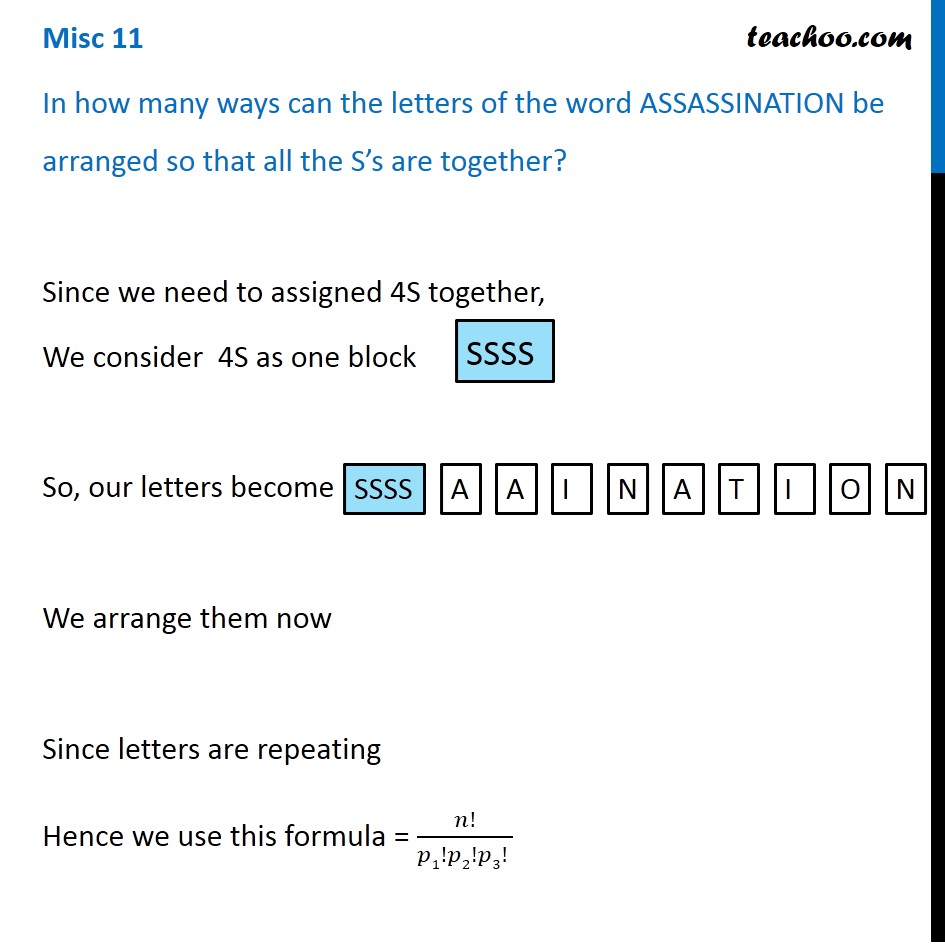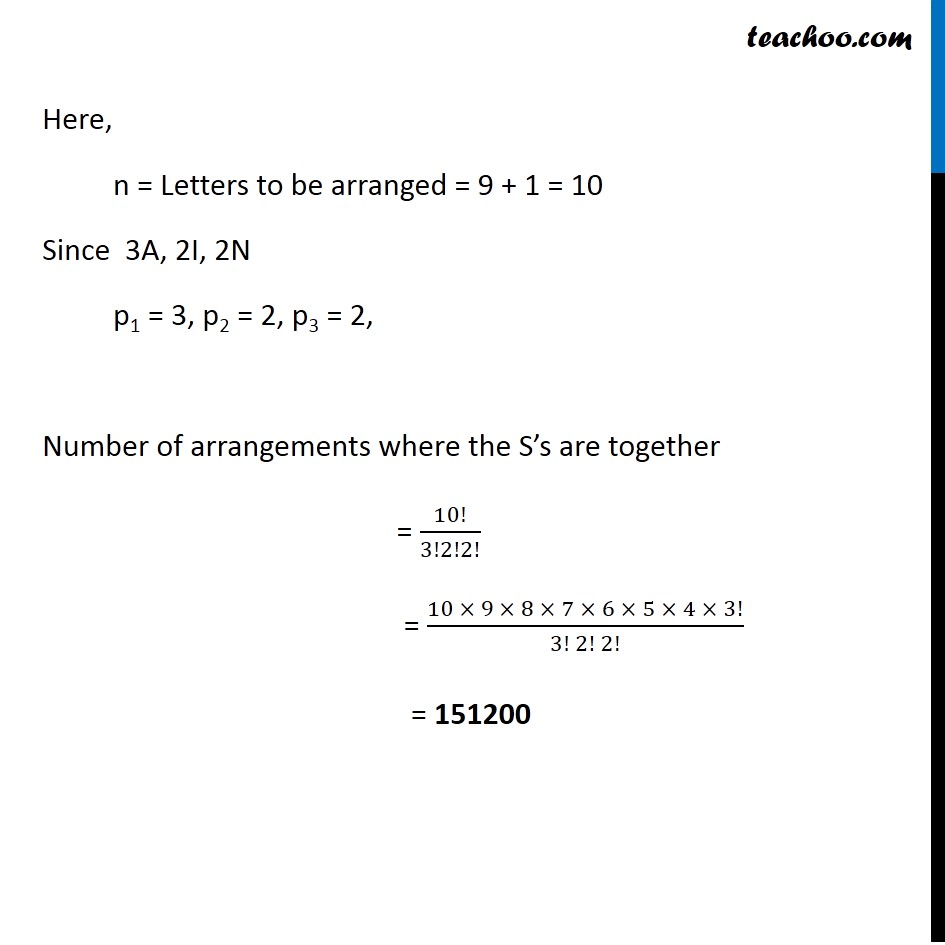1. Class 11
2. Important Questions for exams Class 11
3. Chapter 7 Class 11 Permutations and Combinations

Transcript

Misc 11 In how many ways can the letters of the word ASSASSINATION be arranged so that all the S’s are together? Since we need to assigned 4S together, We consider 4S as one block So, our letters become We arrange them now Since letters are repeating Hence we use this formula = 𝑛!/(𝑝1!𝑝2!𝑝3! ) Here, n = Letters to be arranged = 9 + 1 = 10 Since 3A, 2I, 2N p1 = 3, p2 = 2, p3 = 2, Number of arrangements where the S’s are together = 10!/3!2!2! = (10 × 9 × 8 × 7 × 6 × 5 × 4 × 3!)/(3! 2! 2!) = 151200

Chapter 7 Class 11 Permutations and Combinations

Class 11
Important Questions for exams Class 11